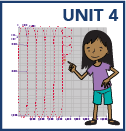## Grade 4

In grade 4 math, your child will:

• understand factors and multiples, e.g., 1,2,4,5,10, and 20 are all factors of 20, and 20 is a multiple of each of those numbers

• understand that a prime number has only 2 factors—1 and itself, while a composite number has more than 2 factors

• multiply multi-digit numbers using strategies based on place value and properties of operations

• divide 2-digit numbers by 1-digit numbers using strategies based on place value and the relationship between multiplication and division

• read, write, and compare multi-digit numbers and round multi-digit numbers to any place

• add and subtract multi-digit numbers accurately and efficiently

• recognize and generate equivalent fractions

• compare two fractions with different numerators and denominators

• add and subtract fractions and mixed numbers with like denominators

• multiply a fraction by a whole number

• write fractions with denominators of 10 or 100 in decimal notation

• compare decimal numbers with digits to the hundredths place

• know the relative sizes of measurement units within one system of units, including metric length, metric mass, customary weight, metric volume, and time

• use formulas for area and perimeter of a rectangle to solve problems

• measure and sketch angles with a protractor

• classify 2-D shapes

• identify and draw lines of symmetry